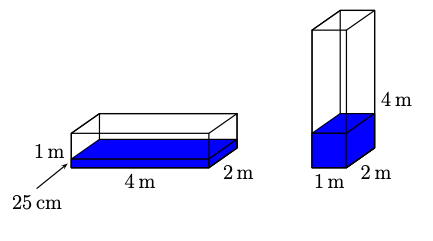Online Math Center

# What Middle Graders Have To Prepare For Math Competitions

Mathematics is introduced to middle school grade through algebra (number systems and number operations), and also through fundamental geometric figures and their features. Any math competition available will test middle graders on the following subjects.

## Algebra and Counting

### Number systems

Mathematics operates, in algebra and geometry, with number systems. There are 6 number systems:

#### Naturals

Indicated by “N”, natural numbers are counting numbers, from 1 to infinity. The whole numbers are all the natural numbers and 0.

The sum and the product of any two natural numbers is a natural number, but not necessarily in subtraction or division.

#### Integers

The integer numbers are the set of real numbers consisting of the natural numbers, their additive inverses and 0. The set of integers is indicated by “J” or “Z”,

The sum, product, and difference of any two integers is also an integer, except division.

#### Rationals

These are the numbers which can be expressed as a fraction between two integers. It will have the form of a fraction: ab or a ratio a:b.

Any of the four basic operations can result in a rational number if we don’t divide them by 0. Any number divided by 0 is not defined. All decimals are also rational.

#### Irrationals

At the opposite corner, there are irrational numbers. These are the numbers that cannot be written as a fraction or ratios.

Some famous irrational numbers are polynomial equations, golden ratios, and transcendental numbers, such as 𝝿 and e

#### Reals

Real numbers are all rational and irrational numbers, thus all the numbers on a line. There is a countable infinity, and an uncountable infinity, most commonly used in set theory.

#### Complex

Going one step further, complex numbers are indicated by the following set:

a + bi, where a and b are real numbers, and ”i” represents the iota number, an imaginary unit.

There are even more complex numbers, known as quaternions, but these are hyper-complex numbers and are not used in math competitions for middle graders.

In order to succeed at math competitions, students have to master the number systems and the four basic operations, since these are used fundamentally in creating more complicated mathematical calculus.

## Circles and Triangles

At the basis of geometry, students should be familiar with the most common shapes, such as the characteristics of a line

#### The geometry of Circles

When it comes to the study of circles and solving geometrical problems, math competitions usually refer to angle-based exercises. Middle school students should learn about: :

• Classification of circles
• Measurement of circles
• Line, rays, and segments of a circle
• Angles, Arcs, Sectors
• Circle Theorems

#### The geometry of Triangles

The most common geometrical problems in math competitions include triangles. So, refine your knowledge of triangles by focusing on:

• Classification of triangles
• Triangle inequalities
• Parts and area of a triangle
• The Pythagorean Theorem
• Congruency of triangles
• Basic trigonometry

It’s recommended that middle graders don’t stop only at circles and triangles. One example of a geometric question at a math competition called Math Kangaroo, for middle school students, in 2022, was:

A water tank with a rectangular base has the dimensions 1 m×2 m×4 m. It contains water to a depth of 25 cm, as shown in the first picture. The tank is turned so that a 1 m × 2 m face becomes the base, as shown in the second picture. What is the depth of the water now?

(A) 25 cm    (B) 50 cm     (C) 75 cm         (D) 1 m         (E) 1.25 m

Image:This shows us that math competitions for middle school students also include exercises on volume, and calculating parts of various geometric figures: square, rectangle, circle, triangle, and trapezoid (polygons).

## At the Online Math Center…

We make math competitions easier by providing tailored individual tutoring with an emphasis on understanding the logic between math operations and applying them from simple to more complex situations. We have a wide range of programs dedicated to studying mathematics, so reach out to us and start your math training.

## Step One

It only takes two steps to schedule a free lesson with an OMC representative.

To get started, please tell us who you are:

## Dear Parent!

Thank you for placing your trust in us to educate your child!

Congratulations on joining the Online Math Center!
See you in class.

## Thank you!

Our manager will be in contact with you shortly.

Please check your inbox for more information. If there is no message from us, please check your email’s “Spam” folder.

## Step Two

Let us know how to contact you. One of our representatives will get back to you shortly.

## Step Two

Awesome! We need to get in touch with your parent or guardian for further discussion. Please check in with them before filling out the form below with their information.

## Step One

It only takes two steps to schedule an appointment with an OMC representative.

To get started, please tell us who you are: